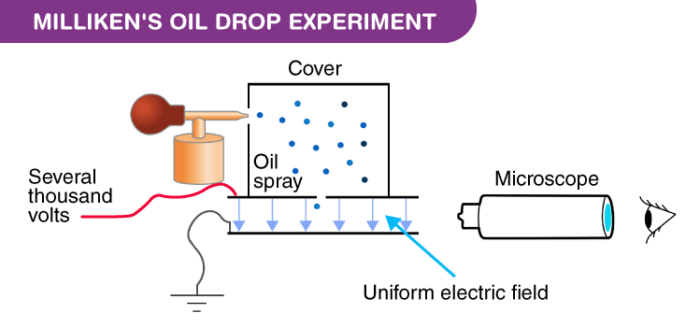# Milliken's Oil Drop Experiment

The Millikens Oil Drop Experiment was an experiment performed by Robert A. Millikan and Harvey Fletcher in 1909 to measure the charge of an electron. This experiment proved to be very crucial in the physics community.

## Millikens Oil Drop Experiment Definition

In the experiment, Milliken allowed charged tiny oil droplets to pass through a hole into an electric field. By varying the strength of the electric field the charge over an oil droplet was calculated, which always came as an integral value of ‘e.’## Apparatus of the Milliken’s Oil Drop Experiment

The apparatus for the experiment was constructed by Milliken and Fletcher. It incorporated two metal plates held at a distance by an insulated rod. There were four holes in the plate, out of which three were there to allow light to pass through them and one was there to allow viewing through the microscope.

Ordinary oil wasn’t used for the experiment as it would evaporate by the heat of the light and so could cause an error in the Millikens Oil Drop Experiment. So, the oil that is generally used in a vacuum apparatus which is of low vapour pressure was used.

## Milliken’s Oil Drop Experiment Procedure

• Oil is passed through the atomizer from where it came in the form of tiny droplets. The pass the droplets through the holes present in the upper plate of the apparatus.
• The downward motions of droplets are observed through a microscope and the mass of oil droplets, then measure their terminal velocity.
• The air inside the chamber is ionized by passing a beam of X-rays through it. The electrical charge on these oil droplets is acquired by collisions with gaseous ions produced by ionization of air.
• The electric field is set up between the two plates and so the motion of charged oil droplets can be affected by the electric field.
• Gravity attracts the oil in a downward direction and the electric field pushes the charge upward. The strength of the electric field is regulated so that the oil droplet reaches an equilibrium position with gravity.
• The charge over the droplet is calculated at equilibrium, which is dependent on the strength of the electric field and mass of droplet.

## Milliken’s Oil Drop Experiment Calculation

Fup = Fdown

Fup = Q . E

Fdown = m.g

is an electron’s charge, is the electric field, is the droplet’s mass, and is gravity.

$$Q\cdot E = m \cdot g$$

Q = $$\frac{m\cdot g}{E}$$

One can see how an electron charge is measured by Millikan. Millikan found that all drops had charges that were 1.6x 10-19 C multiples.

## Milliken’s Oil Drop Experiment Conclusion

The charge over any oil droplet is always an integral value of e (1.6 x 10-19). Hence, the conclusion of  Millikens Oil Drop Experiment is that the charge is said to be quantized, i.e. the charge on any particle will always be an integral multiple of e.

## Frequently Asked Questions – FAQs

### What did Millikan’s oil drop experiment measure?

Millikan oil-drop test, the first simple and persuasive electrical charge calculation of a single electron. It was first conducted by the American physicist Robert A. in 1909. He discovered that all the drops had charges that were simple multiples of a single integer, the electron’s fundamental charge.

### What is the importance of Millikan’s oil drop experiment?

The experiment with Millikan is important since it defined the charge on an electron. Millikan used a very basic, very simple system in which the behaviour of gravitational, electrical, and (air) drag forces were controlled.

### What did Millikan conclude after performing his oil drop experiment?

An integral multiple of the charge on an electron is the charge on every oil decrease. About an electric force. In a relatively small amount, the charge and mass of the atom must be condensed.

### Why charges are quantized?

Charges are quantized since every object’s charge (ion, atom, etc.) Charge quantization, therefore, implies that no random values can be taken from the charge, but only values that are integral multiples of the fundamental charge (proton / electron charge).

### Can charge be created or destroyed?

The Charge Conservation Law does not suggest that it is difficult to generate or remove electrical charges. It also means that any time a negative electrical charge is produced, it is important to produce an equal amount of positive electrical charge at the same time so that a system’s overall charge does not shift.